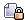Author Topic: Calculation of payment fees  (Read 5816 times)

MilboCalculation of payment fees
« on: April 14, 2014, 12:14:42 pm »
Sometimes people think there is a bug in the calculation of payment fees.

Assume you need \$100 and they want 5% as fee. Then you must actually borrow \$105 and you have to pay fee for the \$5 again => 100 +5 + 0.25 + 0.0125 + 0.00625 + 0.0003125 + ... = ~ 105,2690625....
We use a bit simplified formular and get 105.2631

It sounds first quite reasonable that a fee of 5% and a payment of 100 results in 105. But the The fee is not just x + % of x. Because x + % of x does not consider that the amount to pay increased by 5 and that you have to pay for this 5 again a fee of 5%. The calculation looks like this then => 100 +5 + 0.25 + 0.0125 + 0.000625 + 0.00003125 + ... = ~ 105,26315625.....

This is a geometric progression http://en.wikipedia.org/wiki/Geometric_progression. We use the direct formula

\$cartTotalAmount = (\$cartTotalAmountOrig + \$method->cost_per_transaction) / (1 -(\$method->cost_percent_total * 0.01));

and get  (100 + 0.0) / (1 - (5 * 0.01)) = 105.263157894737
I should fix your bug, please support the VirtueMart project and become a member
______________________________________
Extensions approved by the core team: http://extensions.virtuemart.net/